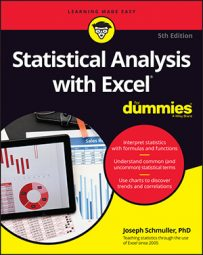##### Statistical Analysis with Excel For DummiesThe worksheet function `F.TEST` calculates an F-ratio on the data from two samples. It doesn’t return the F-ratio. Instead, it provides the two-tailed probability of the calculated F-ratio under H0. This means that the answer is the proportion of area to the right of the F-ratio, and to the left of the reciprocal of the F-ratio (1 divided by the F-ratio).

The image below shows the presented data. It also shows the Function Arguments dialog box for `F.TEST`.

1. Enter the data for each sample into a separate data array and select a cell for the answer. For this example, the data for the Machine 1 sample are in column B and the data for the Machine 2 sample are in column D.
2. From the Statistical Functions menu, select F.TEST to open the Function Arguments dialog box for `F.TEST`.
3. In the Function Arguments dialog box, enter the appropriate values for the arguments. In the Array1 box, enter the sequence of cells that holds the data for the sample with the larger variance. In this example, the Machine 1 data are in B3:B12. In the Array2 box, enter the sequence of cells that holds the data for the other sample. The Machine 2 data are in D3:D17. With values entered for all the arguments, the answer appears in the dialog box.
4. Click OK to put the answer in the selected cell.
The value in the dialog box is greater than .05, so the decision is to not reject H0.

If you had assigned names to those two arrays, the formula in the Formula bar could have been

`=F.TEST(Machine_1,Machine_2)`